# Volume Worksheet – How To Calculate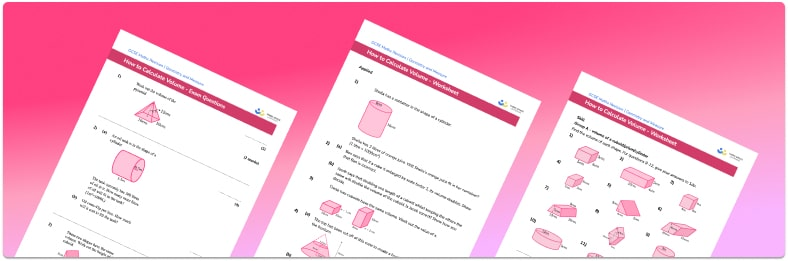• Section 1 of the how to create volume worksheet contains 36 skills-based volume questions, in 3 groups to support differentiation
• Section 2 contains 4 applied volume questions with a mix of worded problems and deeper problem solving questions
• Section 3 contains 4 foundation and higher level GCSE exam style volume questions
• Answers and a mark scheme for all volume questions are provided
• Questions follow variation theory with plenty of opportunities for students to work independently at their own level
• All questions created by fully qualified expert secondary maths teachers
• Suitable for GCSE maths revision for AQA, OCR and Edexcel exam boards

• This field is for validation purposes and should be left unchanged.

You can unsubscribe at any time (each email we send will contain an easy way to unsubscribe). To find out more about how we use your data, see our privacy policy.

### Volume at a glance

The volume of a 3D shape is the amount of space enclosed inside it. The units of measurement for volume are cubic units e.g. cm3, m3, mm3. A simple introduction to calculating volume is to use manipulatives to physically fill a 3D shape with unit cubes, then work out the volume by counting cubes.

The simplest volume formulae are for volume of a cube and volume of a cuboid; we multiply length, width and height together.

We can also work out the volume of prisms. A prism is a geometric shape with flat faces that has a constant cross-section. An example of a prism is a cuboid (known as rectangular prism), because the cross-section of a cuboid is a rectangle. Working out the volume of a rectangular prism in this way leads to the general formula for any prism: area of cross section length of the prism.

To work out the volume of other prisms, such as triangular prisms, it is important that students are confident with area formulae. A cylinder is not a prism, because it has curved faces, but the principle for working out the volume of cylinders is the same, calculate the area of the circular face, then multiply by the length or height of the cylinder.

There are also formulae for volume of cones, volume of pyramids and volume of spheres which are given in GCSE exams.

Students are also expected to work out the volume of compound shapes. A compound shape is made up of two or more simple 3D shapes. These may be presented as word problems or relating to real life scenarios, such as the volume of a storage silo made from a cylinder and a cone.

Care must be taken when working with decimals and students must only round their answers when they have completed the question.

Looking forward, students can then progress to additional geometry worksheets, for example a how to work out surface area printable worksheet or volume of prisms worksheet.For more teaching and learning support on Geometry our GCSE maths lessons provide step by step support for all GCSE maths concepts.

## Related worksheets

Congruent Triangles Worksheet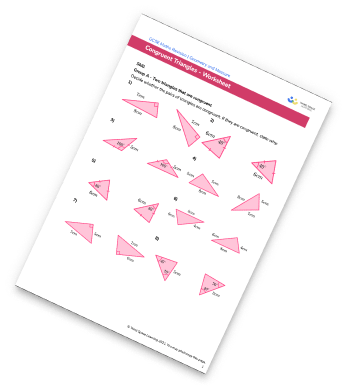3D Shapes Worksheet Vertices, Edges, Faces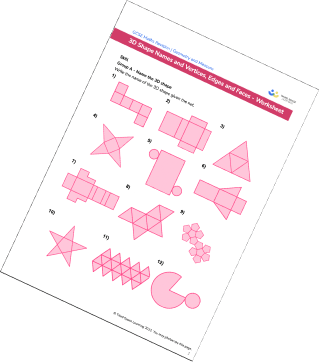Transformations Worksheets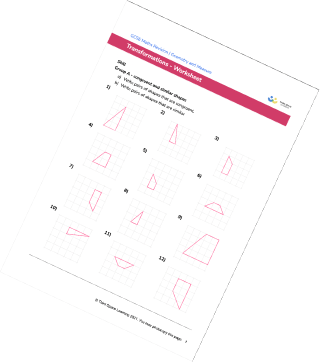Translating Shapes Worksheet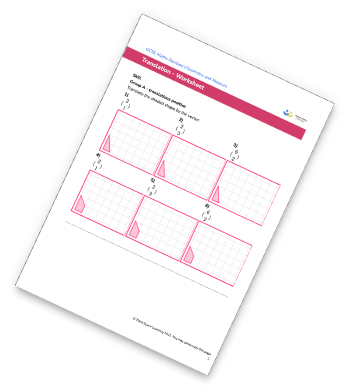## Do you have KS4 students who need more focused attention to succeed at GCSE?There will be students in your class who require individual attention to help them succeed in their maths GCSEs. In a class of 30, it’s not always easy to provide.

Help your students feel confident with exam-style questions and the strategies they’ll need to answer them correctly with our dedicated GCSE maths revision programme.

Lessons are selected to provide support where each student needs it most, and specially-trained GCSE maths tutors adapt the pitch and pace of each lesson. This ensures a personalised revision programme that raises grades and boosts confidence.

Find out more